﻿ 人民币离岸市场与在岸市场的联动关系研究 The Study on Interactions between RMB Offshore and Onshore Markets

Finance
Vol. 09  No. 05 ( 2019 ), Article ID: 32242 , 15 pages
10.12677/FIN.2019.95061

The Study on Interactions between RMB Offshore and Onshore Markets

Shuorong Huang, Yuping Lan

International Business Faculty, Zhuhai Campus, Beijing Normal University, Zhuhai Guangdong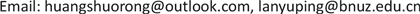Received: Aug. 29th, 2019; accepted: Sep. 13th, 2019; published: Sep. 20th, 2019ABSTRACT

In recent years, with RMB inclusion in SDR and the rising of China’s comprehensive power, RMB internationalization strategy is advancing. By carrying out the cross-border RMB settlement, the RMB now as the settlement currency is widely accepted by the overseas entities. In the processing of RMB internationalization, Hong Kong RMB offshore market has become the most important RMB offshore markets. In the long run, the development and establishment of offshore markets could increase credibility abroad, lay out a solid foundation for the RMB to become international reserve currency and deepen reform of financial system in onshore markets. Therefore, it will be great practical to study the interaction effect of two markets and their influencing factors. Based on the Interest parity theory, the paper analyzes the channel of RMB flow and arbitrage and hedging model. By making use of Granger causality test and the VAR model, the paper studies the dynamic changes between the interest rates and exchange rates. The empirical results show that in terms of the interest rates, there is a faint relationship between two markets in short terms. The price information is mainly transmitted from the offshore market to the onshore market; Off shore market may be the pricing center of RMB. According to empirical results, the paper suggests to boost market-based reform of interest rate and exchange rate and to accelerate the opening of capital account, to expand the circulation of offshore markets and onshore markets.

Keywords:RMB, Offshore Market, Onshore Market1. 引言

2016年以来，中国经济结构不断优化，国际上人民币在世界范围内展露锋芒，以人民币计价的交易数量在国际债券与票据、国际信贷、直接投资等领域上涨迅速。人民币作为贸易结算货币和投资货币的职能不断增强，作为储备货币的功能逐渐显现。根据环球银行金融电信协会(SWIFT)数据显示，截至2019年1月，人民币在全球支付中的份额上升至2.15%，排名居第5位。目前，离岸人民币市场已形成以中国香港为主，伦敦、新加坡、欧洲及东南亚地区多点并行的格局。得益于离岸市场的快速发展，人民币跨境收付总体平衡，境外主体持有境内人民币资产的意愿不断提升，人民币外汇交易与货币互换范围不断扩大，人民币跨境制度系统趋于完善，境外人民币业务清算行的数量增加。同时人民币产品不断多元化，如内地香港基金互认、“沪伦通”、“沪港通”、“深港通”和“债券通”等一系列新制度安排推出，进一步打通离岸和在岸的流动渠道，不断活跃离岸人民币市场。

2. 文献综述

3. 理论分析与模型

3.1. 离岸人民币市场概述

3.2. 离岸与在岸市场资金联动机制分析

3.2.1. 人民币资金流动渠道分析

3.2.2. 套利套汇机制分析

3.3. 离岸市场与在岸市场相互影响关系分析

3.3.1. 人民币离岸市场对在岸市场的有利影响

3.3.2. 人民币离岸市场对在岸市场的不利影响

3.3.3. 人民币在岸市场对离岸人民币的影响

3.4. 实证分析方法与模型

3.4.1. 计量研究方法

3.4.2. VAR模型

VAR模型的表达方式为：

${y}_{t}=\alpha +{\sum }_{i=1}^{p}{\beta }_{i}{y}_{t-i}+{\epsilon }_{t}$ (1)

${y}_{t}=\left[\begin{array}{c}\text{SHIBOR},t\\ \text{HIBOR},t\end{array}\right],\alpha =\left[\begin{array}{c}{\alpha }_{1}\\ {\alpha }_{2}\end{array}\right],\beta =\left[\begin{array}{c}{\beta }_{11},i,{\beta }_{12},i\\ {\beta }_{21},i,{\beta }_{22},i\end{array}\right],\epsilon =\left[\begin{array}{c}{\epsilon }_{1t}\\ {\epsilon }_{2t}\end{array}\right],{\epsilon }_{it}\sim N\left(0,{\sigma }^{2}\right)$ (2)

${y}_{t}=\left[\begin{array}{c}\text{CNY},t\\ \text{CNH},t\end{array}\right],\alpha =\left[\begin{array}{c}{\alpha }_{1}\\ {\alpha }_{2}\end{array}\right],\beta =\left[\begin{array}{c}{\beta }_{11},i,{\beta }_{12},i\\ {\beta }_{21},i,{\beta }_{22},i\end{array}\right],\epsilon =\left[\begin{array}{c}{\epsilon }_{1t}\\ {\epsilon }_{2t}\end{array}\right],{\epsilon }_{it}\sim N\left(0,{\sigma }^{2}\right)$ (3)

${y}_{t}=\left[\begin{array}{c}\text{CNYDF},t\\ \text{NDF},t\end{array}\right],\alpha =\left[\begin{array}{c}{\alpha }_{1}\\ {\alpha }_{2}\end{array}\right],\beta =\left[\begin{array}{c}{\beta }_{11},i,{\beta }_{12},i\\ {\beta }_{21},i,{\beta }_{22},i\end{array}\right],\epsilon =\left[\begin{array}{c}{\epsilon }_{1t}\\ {\epsilon }_{2t}\end{array}\right],{\epsilon }_{it}\sim N\left(0,{\sigma }^{2}\right)$ (4)

${y}_{t}=\left[\begin{array}{c}\text{LC},t\\ \text{HC},t\\ \text{FW},t\end{array}\right],\alpha =\left[\begin{array}{c}{\alpha }_{1}\\ {\alpha }_{2}\\ {\alpha }_{3}\end{array}\right],\beta =\left[\begin{array}{c}{\beta }_{11},i,{\beta }_{12},i,{\beta }_{13},i\\ {\beta }_{21},i,{\beta }_{22},i,{\beta }_{23},i\\ {\beta }_{31},i,{\beta }_{32},i,{\beta }_{33},i\end{array}\right],\epsilon =\left[\begin{array}{c}{\epsilon }_{1t}\\ {\epsilon }_{2t}\\ {\epsilon }_{3t}\end{array}\right],{\epsilon }_{it}\sim N\left(0,{\sigma }^{2}\right)$ (5)

3.4.3. 样本选取与数据说明

4. 人民币离岸市场与在岸市场联动关系实证分析

4.1. 离岸与在岸市场利率联动关系分析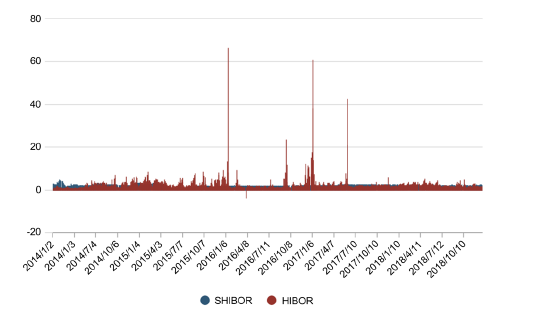Figure 1. SHIBOR and HIBORTable 1. Granger causality test results of SHIBOR and HIBOR

4.2. 离岸与在岸市场即期汇率联动关系分析

4.2.1 . 走势图分析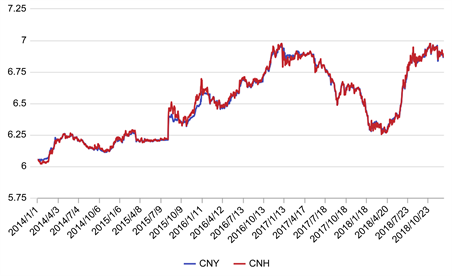Figure 2. CNY and CNH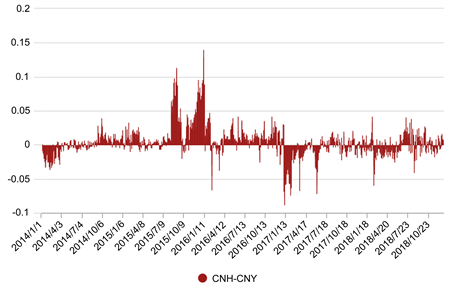Figure 3. The spread of CNY and CNH

4.2.2. 数据描述性统计分析Table 2. Descriptive statistics analysis results of CNY and CNH

4.2.3. 平稳性检验与格兰杰因果检验Table 4. Granger causality test results of CNY and CNH

4.2.4. 基本的VAR模型

*Indicates lag order selected by the criterion.

Table 6. Vector autoregression estimates

Standard errors in ( ) & t−statistics in [ ].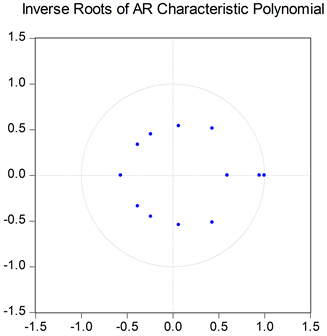Figure 4. VAR(6) stability tests

4.2.5. 脉冲响应函数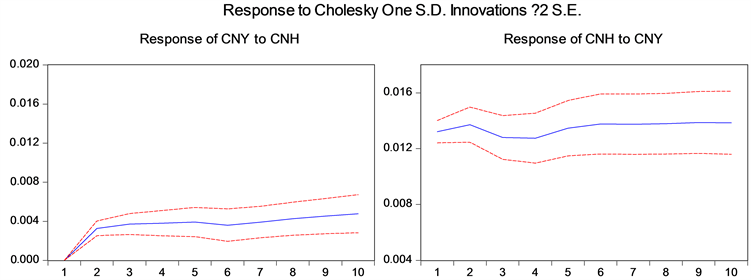Figure 5. Impulse-response function of CNY and CNH

4.2.6. 方差分解Table 7. Variance decomposition results of CNY and CNH

4.3. 离岸与在岸市场远期汇率联动关系分析

4.3.1. 研究背景与走势图分析

2018年初，国家外汇管理局对在岸市场的远期结售汇业务放开差额交割，境内NDF市场成型，完全实现市场化，有利于境内外汇套期保值的业务推进。而由于境内存在外汇管制，在岸市场与离岸市场的传导机制尚未被完全打通，远期结售汇业务上产生的差价在一定程度上进入境内受限，而境外NDF能够在一定程度上弥补风险，因此研究境外NDF和在岸远期汇率之间的关系存在意义。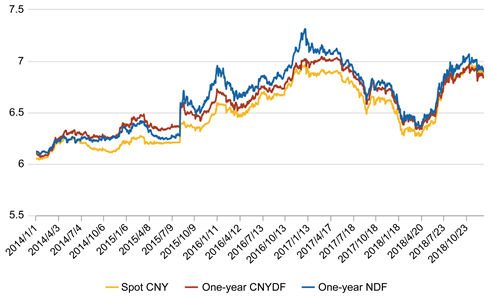Figure 6. CNYDF12M, NDF and Spot CNY

4.3.2. 数据描述性统计分析与格兰杰因果检验Table 8. Descriptive statistics analysis results of NDF and CNYDF

4.3.3. 格兰杰因果检验Table 9. Granger causality test results of NDF and CNYDF

4.3.4. 脉冲响应分析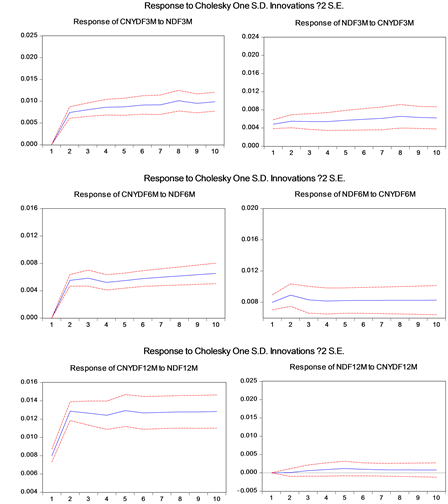Figure 7. Impulse-response function of CNYDF and NDF

4.4. 离岸与在岸市场利差、汇差联动关系分析

4.4.1. 研究原因与变量设定

4.4.2. 格兰杰因果检验Table 10. Granger causality test results of LC, HC and FW

4.4.3. 脉冲响应函数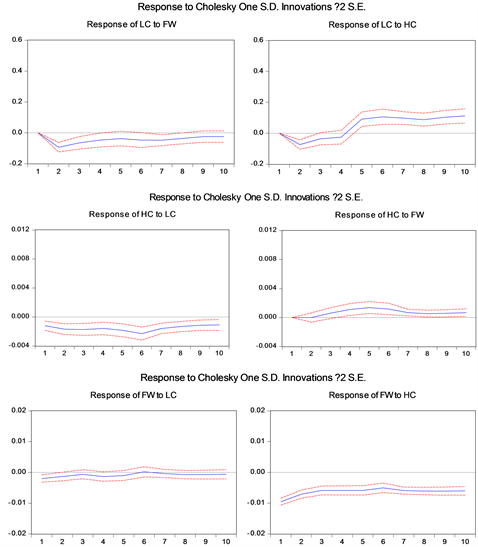Figure 8. Impulse-response function of LC, HC and FW

5. 结论与政策建议

5.1. 结论与说明

5.2. 政策建议

5.2.1. 加快在岸市场利率汇率市场化改革与资本可自由兑换进程

5.2.2. 增强在岸人民币外汇市场的定价权和风险防范能力

5.2.3. 建立以香港为核心的人民币离岸市场体系

The Study on Interactions between RMB Offshore and Onshore Markets[J]. 金融, 2019, 09(05): 542-556. https://doi.org/10.12677/FIN.2019.95061

1. 1. 巴曙松, 郭云钊, KVB昆仑国际离岸金融项目组. 离岸金融市场发展研究——国际趋势与中国路径[M]. 北京: 北京大学出版社, 2008.

2. 2. 杨承亮. 人民币离岸市场与在岸市场联动关系研究[D]: [博士学位论文]. 北京: 中国社会科学院研究生院, 2014.

3. 3. 屠婕. 在岸与香港离岸人民币外汇市场联动效应及其影响因素的研究[D]: [硕士学位论文]. 南京: 南京大学, 2018.

4. 4. 张明, 何帆. 人民币国际化进程中在岸离岸套利现象研究[J]. 国际金融研究, 2012(10): 47-54.

5. 5. 张斌, 徐奇渊. 汇率与资本项目管制下的人民币国际化[J]. 国际经济评论, 2012(4): 63-72.

6. 6. 乔依德, 李蕊, 葛佳飞. 人民币国际化: 离岸市场与在岸市场的互动[J]. 国际经济评论, 2014(2): 93-102.

7. 7. 戎如香. 人民币在岸远期市场和离岸NDF市场关系的实证研究[J]. 当代财经, 2009(1): 53-58.

8. 8. 龙艺, 胡静. 人民币离岸与在岸市场交互影响分析——基于格兰杰和向量误差修正模型分析[J]. 中共南京市委党校学报, 2018(3): 33-42.

9. 9. Gerber, P. (2011) What Currently Drives CNH Market Equilibrium. The Council on Foreign Relations & China Development Research Foundation Workshop on the International of the Renminbi, Beijing.

10. 10. 任苑. 香港人民币离岸市场的发展及对在岸货币供给和汇率的影响研究[D]: [硕士学位论文]. 成都: 西南财经大学, 2013.